Like   Tweet

## What's Dawn up to this summer? Theater Props!

 table div table+table+table div table,table.module-2{width:31.89%;float:right;padding:0}table div table+table+table div table a{border:0 none;text-decoration:none}table div table+table+table div table img{width:100%!important;border:0 none;text-decoration:none}table div table+table+table div table td{width:100%;padding:0 0 20px 20px}/* styles */ Hi All, The last six weeks I've been working on theater props and set painting for a production of "The Most Happy Fella" which had its opening night this past Friday at the El Cerrito Performing Arts Theater on the campus of ECHS. One more weekend left for a chance to see this amazing show (and my props below!). It's really, really magical when the music, the set, the costumes and the performances all come together.
 table div table+table+table+table div table{width:100%;padding:0}table div table+table+table+table div table img{width:96.23%;padding:0;float:none}table div table+table+table+table div table td{width:100%;padding:0 1.88% 18px}/* styles */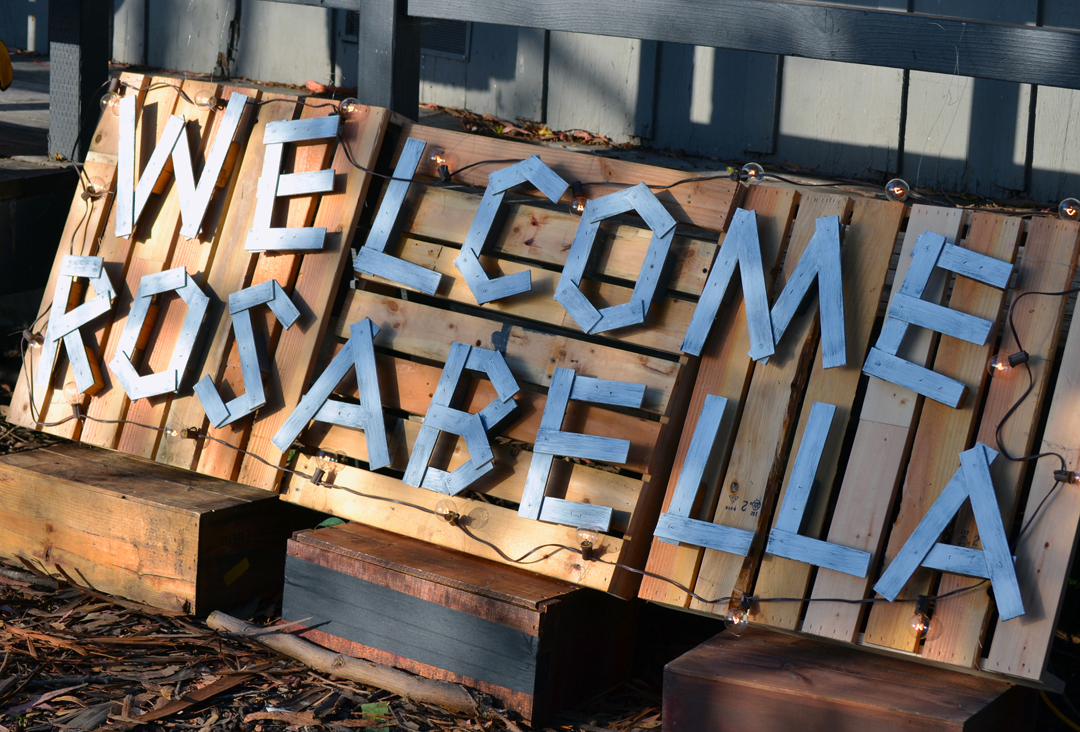/* styles */ The lighted sign needed to look like it was made with materials they would have been around a vineyard. I made the letters from scraps from the last show I worked on. The background was also shop scraps - made to look like pallets. I love the way this turned out!
 table div table+table+table+table+table+table+table div table{width:100%;padding:0}table div table+table+table+table+table+table+table div table img{width:96.23%;padding:0;float:none}table div table+table+table+table+table+table+table div table td{width:100%;padding:0 1.88% 18px}/* styles */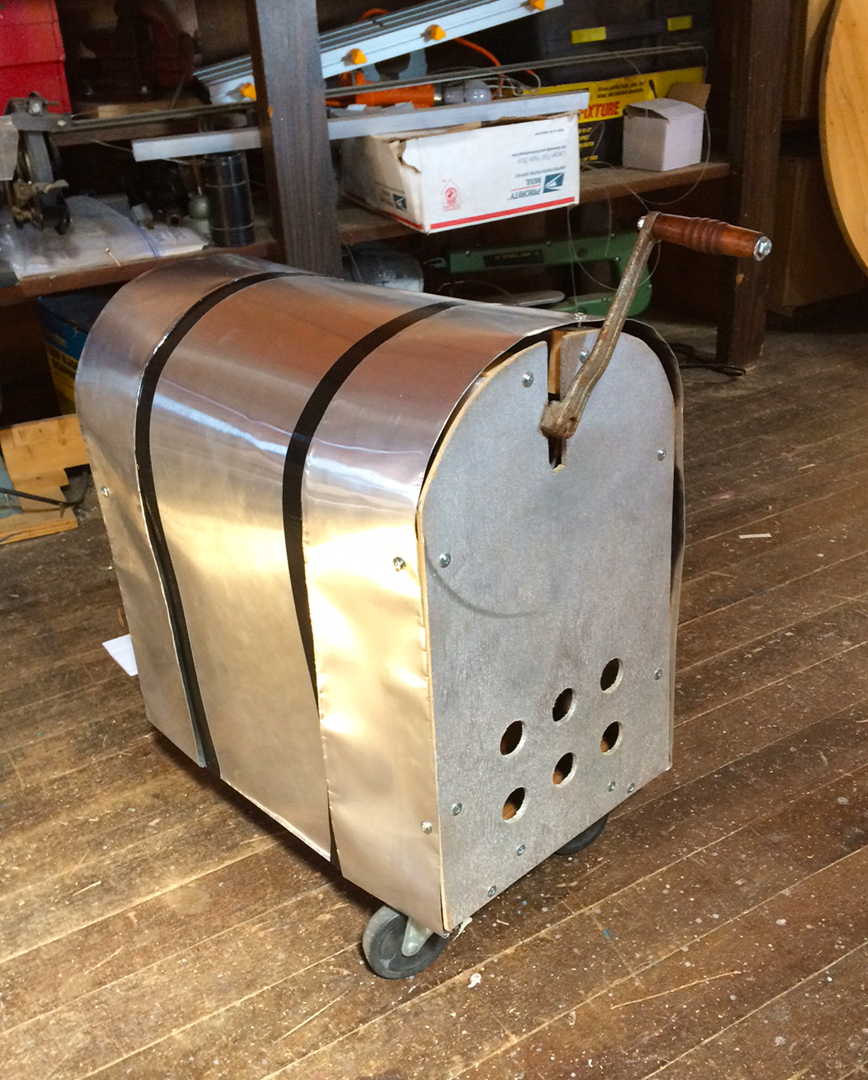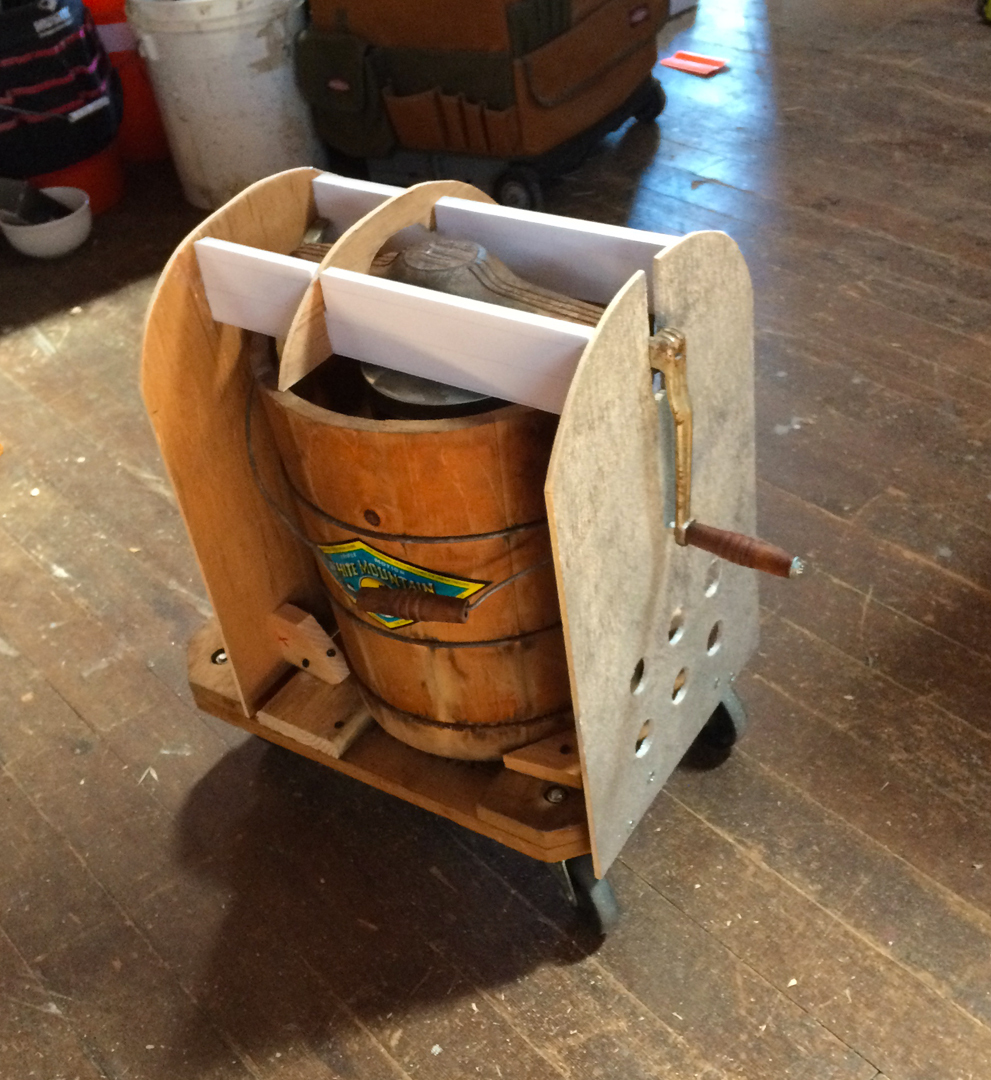/* styles */ I made the hand-cranked generator out of our hand-cranked ice cream maker. I set it on a furniture dolly and covered it in metal flashing. Voila!
 table div table+table+table+table+table+table+table+table+table+table div table{width:100%;padding:0}table div table+table+table+table+table+table+table+table+table+table div table img{width:96.23%;padding:0;float:none}table div table+table+table+table+table+table+table+table+table+table div table td{width:100%;padding:0 1.88% 18px}/* styles */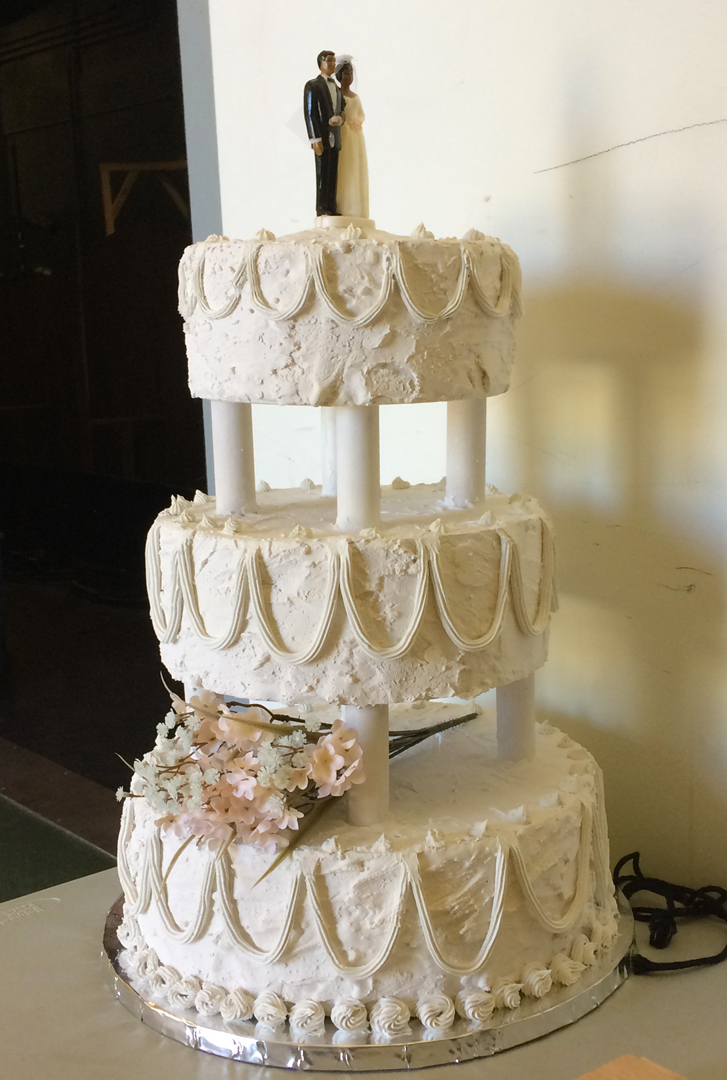/* styles */ For the wedding cake I made the cake shapes out of foamcore. The pillars are wooden dowels. One of our interns Ivy did a great job decorating it with plaster and caulk.
 table div table+table+table+table+table+table+table+table+table+table+table+table+table div table{width:100%;padding:0}table div table+table+table+table+table+table+table+table+table+table+table+table+table div table img{width:96.23%;padding:0;float:none}table div table+table+table+table+table+table+table+table+table+table+table+table+table div table td{width:100%;padding:0 1.88% 18px}/* styles */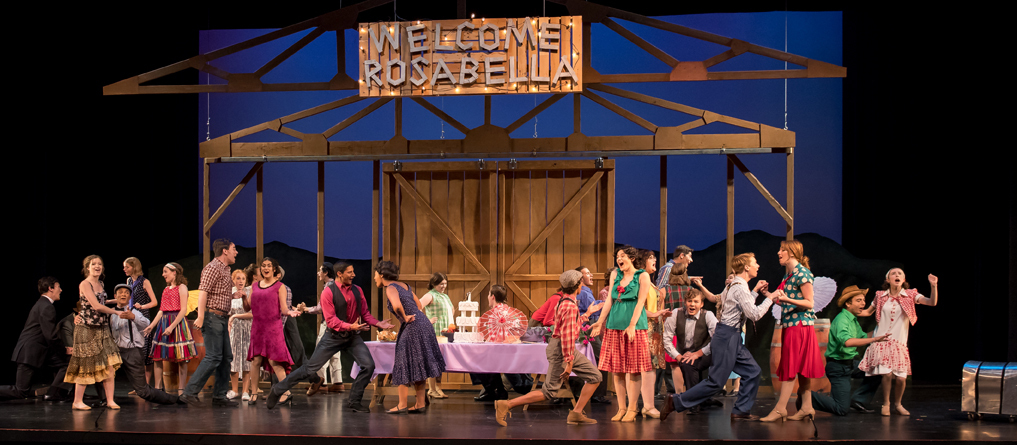/* styles */ Here is a shot from one of the opening scenes where you can see the Rosabella sign, the generator (far right) and the wedding cake (center).
 table div table+table+table+table+table+table+table+table+table+table+table+table+table+table+table+table div table{width:100%;padding:0}table div table+table+table+table+table+table+table+table+table+table+table+table+table+table+table+table div table img{width:96.23%;padding:0;float:none}table div table+table+table+table+table+table+table+table+table+table+table+table+table+table+table+table div table td{width:100%;padding:0 1.88% 18px}/* styles */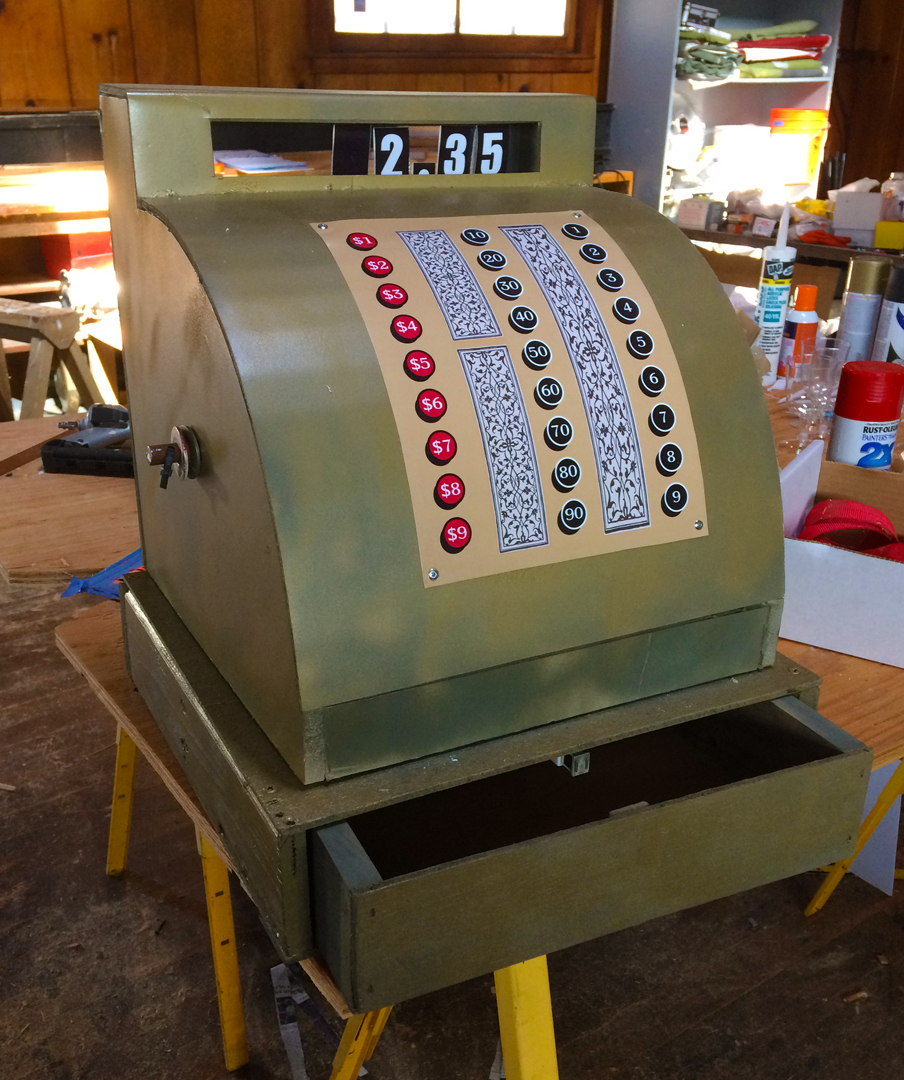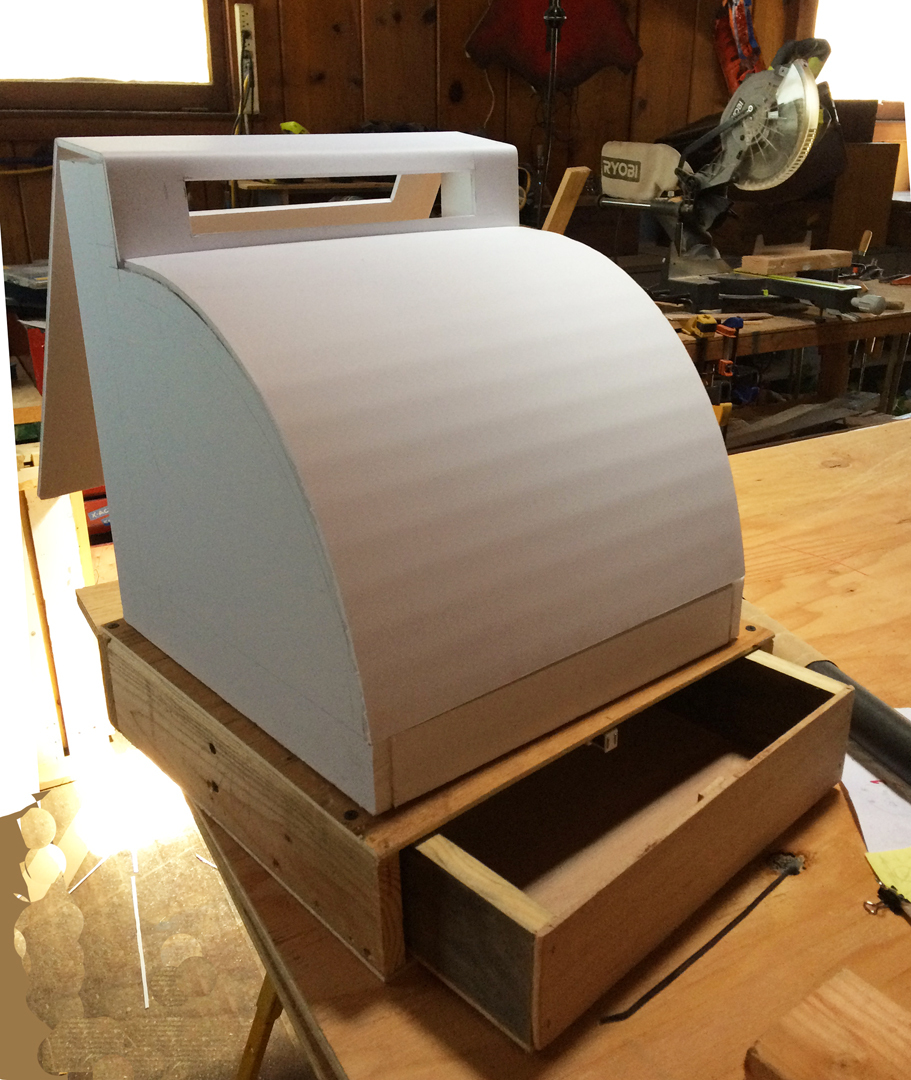/* styles */ The old-timey cash register is made with a wood base. I used foamcore to make the upper rounded face and the window that shows the amount of the sale. There was a random crank laying around the shop so I incorporated that into it too. The panel with the buttons was printed out and spray mounted on.
 table div table+table+table+table+table+table+table+table+table+table+table+table+table+table+table+table+table+table+table div table{width:100%;padding:0}table div table+table+table+table+table+table+table+table+table+table+table+table+table+table+table+table+table+table+table div table img{width:96.23%;padding:0;float:none}table div table+table+table+table+table+table+table+table+table+table+table+table+table+table+table+table+table+table+table div table td{width:100%;padding:0 1.88% 18px}/* styles */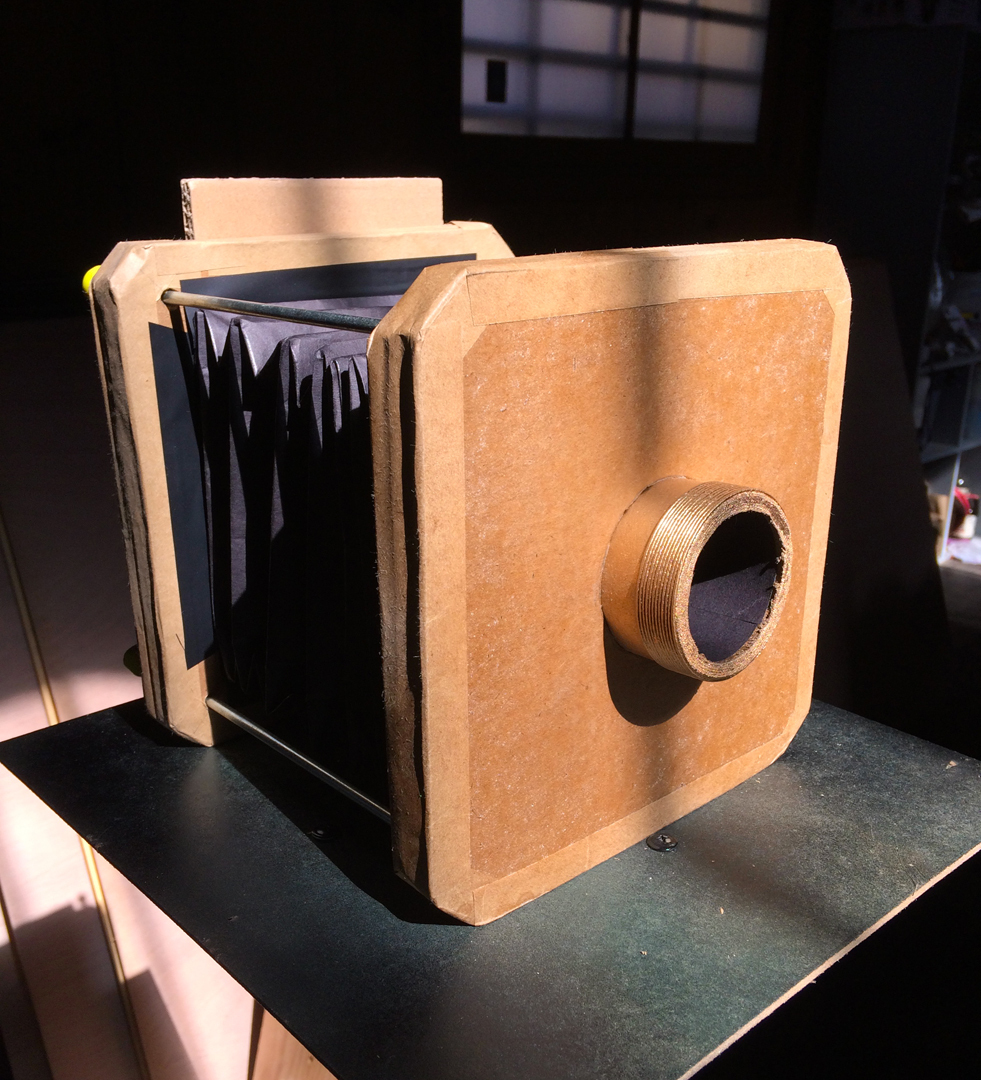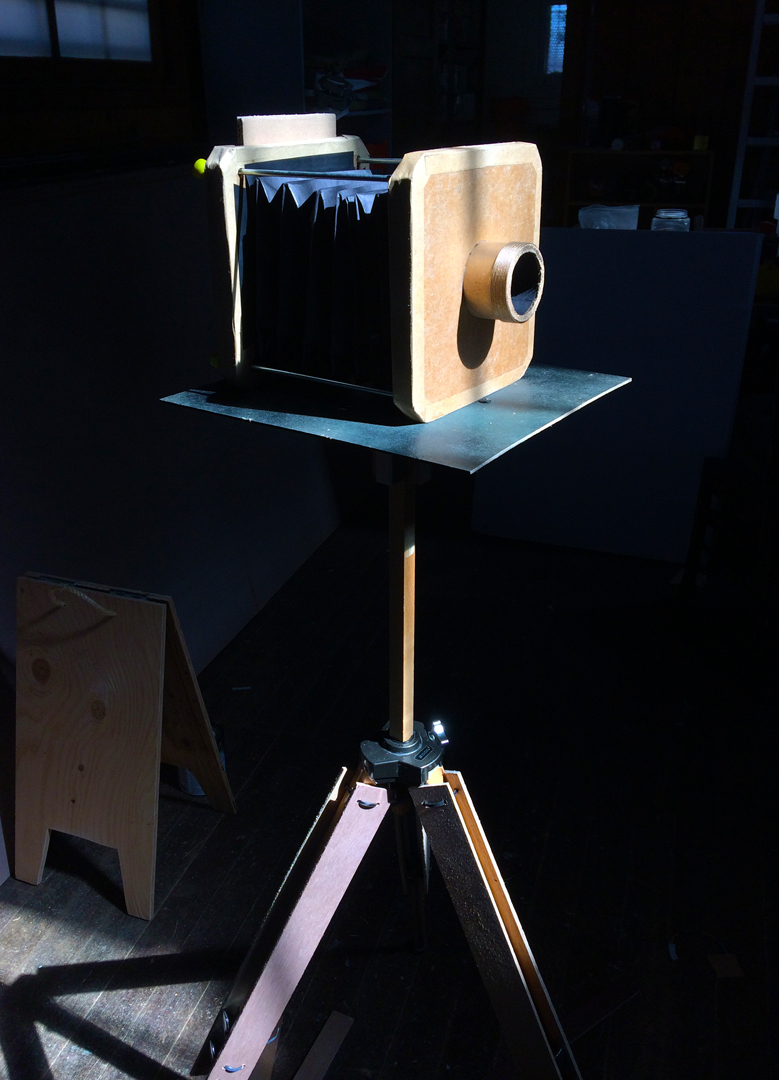/* styles */ The period camera was probably the most challenging. I figured out how to make the bellows out of black paper. I used cardboard, cardboard tube and craft paper tape to form the rest of the camera. Thin metal rods connects the front and back sections and allows them to move. I used my "real" tripod, attached some wood slats and taped the lower legs with brown duct tape. I converted a squeegee handle into the device that holds the flash powder.
 table div table+table+table+table+table+table+table+table+table+table+table+table+table+table+table+table+table+table+table+table+table+table div table{width:100%;padding:0}table div table+table+table+table+table+table+table+table+table+table+table+table+table+table+table+table+table+table+table+table+table+table div table img{width:96.23%;padding:0;float:none}table div table+table+table+table+table+table+table+table+table+table+table+table+table+table+table+table+table+table+table+table+table+table div table td{width:100%;padding:0 1.88% 18px}/* styles */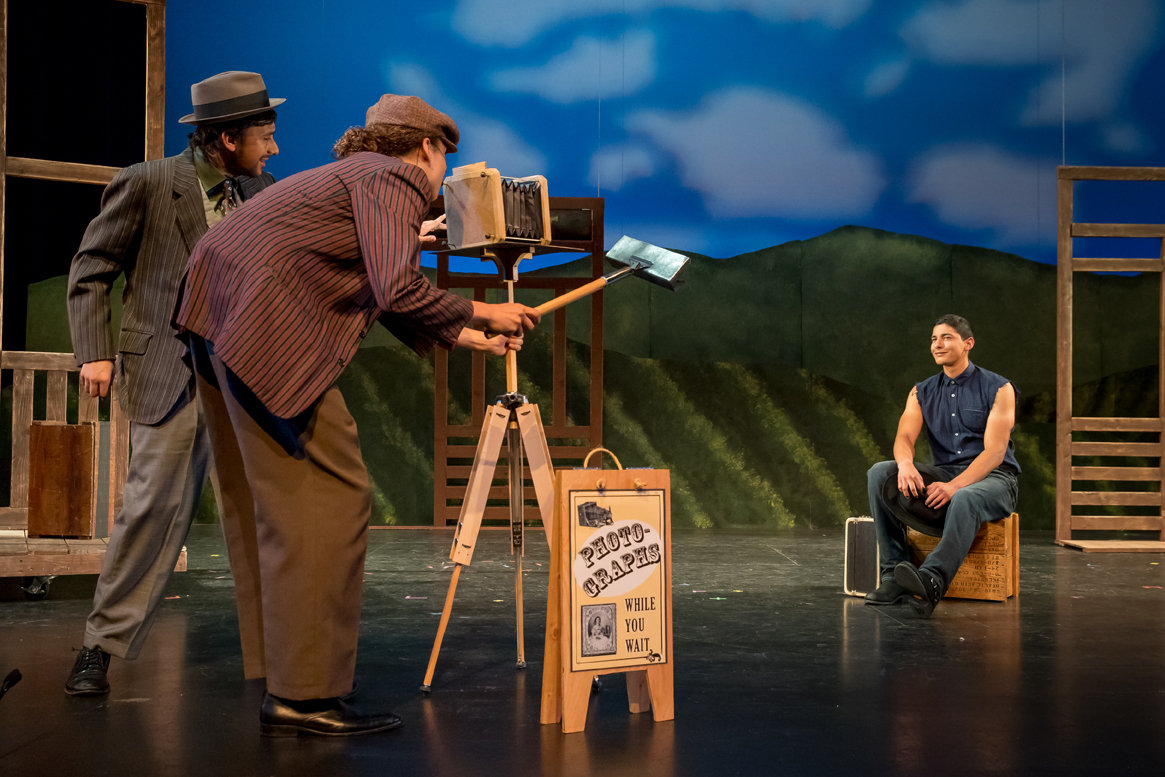table div table+table+table+table+table+table+table+table+table+table+table+table+table+table+table+table+table+table+table+table+table+table+table+table div table{width:100%;padding:0}table div table+table+table+table+table+table+table+table+table+table+table+table+table+table+table+table+table+table+table+table+table+table+table+table div table img{width:96.23%;padding:0;float:none}table div table+table+table+table+table+table+table+table+table+table+table+table+table+table+table+table+table+table+table+table+table+table+table+table div table td{width:100%;padding:0 1.88% 18px}/* styles */## Friday 7/28 is "pay what you want night."

 /* styles */ If tickets prices are keeping you away come to this Friday's performance and "pay what you want!". You won't be disappointed. Last three shows: Friday July 28 at 7pm Saturday July 29 at 7pm Sunday July 30 at 2pm For more information about the show click here. Hope to see you there!! Dawn p.s. To check out more of my work see my website - including deals on my t-shirts (\$5 & \$10)!!